# 1585 is a Centered Triangular Number

### Today’s Puzzle:

What geometric shape can 1585 tiny squares be arranged into?

If you answered a 5 × 317 rectangle, you would be right, but there is another shape that is probably more interesting than that rectangle: 1585 tiny squares can be arranged into a centered triangle as illustrated below.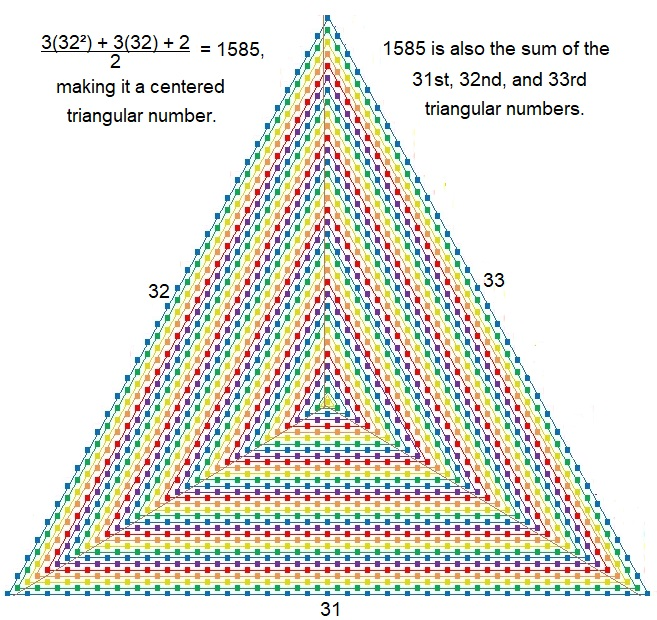Can you see the three consecutive triangular numbers that make up this centered triangle?

### Factors of 1585:

• 1585 is a composite number.
• Prime factorization: 1585 = 5 × 317.
• 1585 has no exponents greater than 1 in its prime factorization, so √1585 cannot be simplified.
• The exponents in the prime factorization are 1 and 1. Adding one to each exponent and multiplying we get (1 + 1)(1 + 1) = 2 × 2 = 4. Therefore 1585 has exactly 4 factors.
• The factors of 1585 are outlined with their factor pair partners in the graphic below.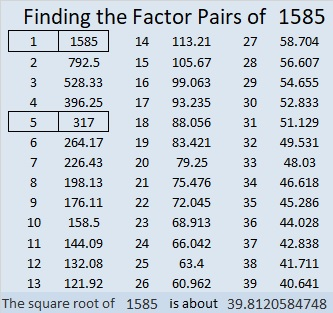### More about the Number 1585:

1585 is the sum of two squares in two different ways:
39² + 8² = 1585
36² + 17² = 1585

1585 is the hypotenuse of FOUR Pythagorean triples:
375-1540-1585, which is 5 times (75-308-317),
624-1457-1585, calculated from 2(39)(8), 39² – 8², 39² + 8²
951-1268-1585, which is (3-4-5) times 317, and
1007-1224-1585, calculated from 36² – 17², 2(36)(17), 36² + 17².

# 31 Flavors of 1396

The first 52 triangular numbers are 0, 1, 3, 6, 10, 15, 21, 28, 36, 45, 55, 66, 78, 91, 105, 120, 136, 153, 171, 190, 210, 231, 253, 276, 300, 325, 351, 378, 406, 435, 465, 496, 528, 561, 595, 630, 666, 703, 741, 780, 820, 861, 903, 946, 990, 1035, 1081, 1128, 1176, 1225, 1275, 1326, 1378.

OEIS.org informs us that 1396 can be written as the sum of three triangular numbers in 31 different ways. It is the smallest number that can make that claim!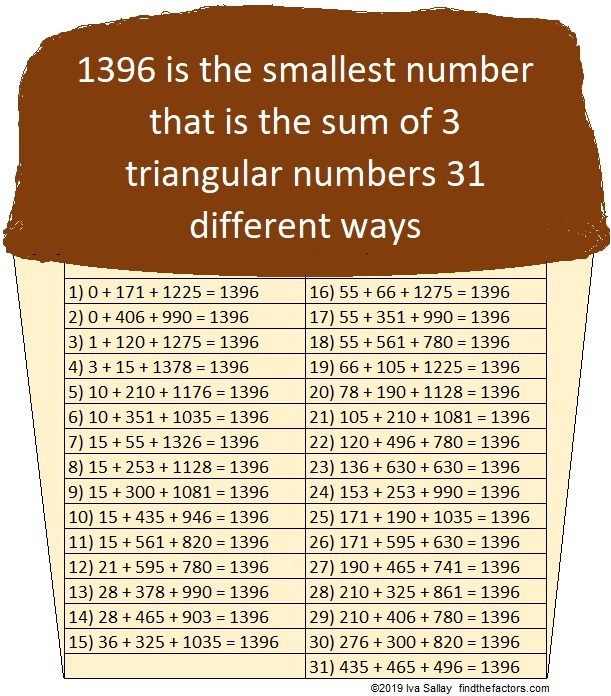That 31st way is written with three consecutive triangular numbers, 435, 465, and 496, which are the 29th, 30th, and 31st triangular numbers respectively. That fact makes 1396 the 31st Centered Triangular Number as well!That is, at least, 1396 is the 31st number on the list. You can also calculate it using this formula: [3(30²) + 3(30) + 2]/2 = 1396

Here’s more about the number 1396:

• 1396 is a composite number.
• Prime factorization: 1396 = 2 × 2 × 349, which can be written 1396 = 2² × 349
• 1396 has at least one exponent greater than 1 in its prime factorization so √1396 can be simplified. Taking the factor pair from the factor pair table below with the largest square number factor, we get √1396 = (√4)(√349) = 2√349
• The exponents in the prime factorization are 2 and 1. Adding one to each exponent and multiplying we get (2 + 1)(1 + 1) = 3 × 2 = 6. Therefore 1396 has exactly 6 factors.
• The factors of 1396 are outlined with their factor pair partners in the graphic below.1396 is the sum of two squares:
36² + 10² = 1396

1396 is the hypotenuse of a Pythagorean triple:
720-1196-1396 calculated from 2(36)(10), 36² – 10², 36² + 10²

# 1306 is a Centered Triangular Number

1306 tiny squares can be arranged in a triangle as I have done in the graphic below. This arrangement makes 1306 a centered triangular number: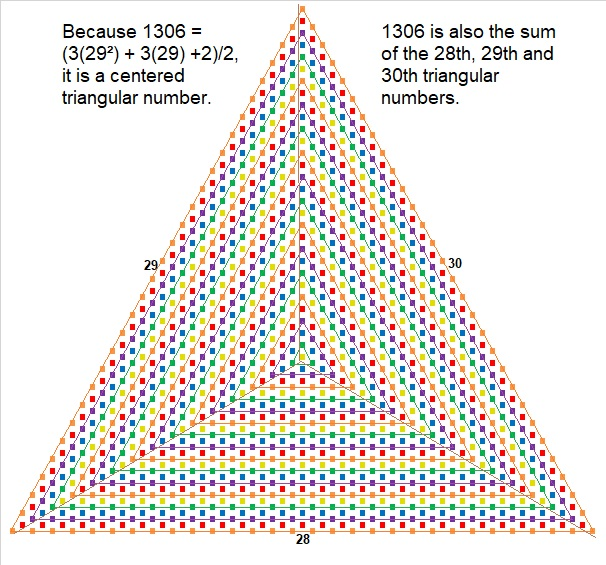Here’s more information about the number 1306:

• 1306 is a composite number.
• Prime factorization: 1306 = 2 × 653
• The exponents in the prime factorization are 1 and 1. Adding one to each and multiplying we get (1 + 1)(1 + 1) = 2 × 2 = 4. Therefore 1306 has exactly 4 factors.
• Factors of 1306: 1, 2, 653, 1306
• Factor pairs: 1306 = 1 × 1306 or 2 × 653
• 1306 has no square factors that allow its square root to be simplified. √1306 ≈ 36.13862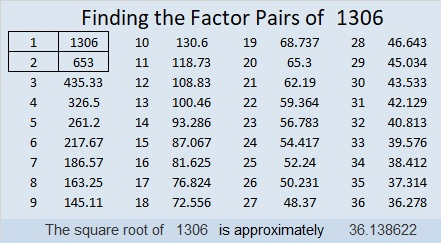OEIS.org informs us that 1306 = 1¹ + 3² + 0³ + 6⁴.

1306 is the sum of two squares:
35² + 9² = 1306

1306 is the hypotenuse of a Pythagorean triple:
630-1144-1306 calculated from 2(35)(9), 35² – 9², 35² + 9²

# 1219 is a Centered Triangular Number

If you look at an ordered list of centered triangular numbers, 1219 will be the 29th number on the list.

Study this graphic to see why:Here’s more about the number 1219:

• 1219 is a composite number.
• Prime factorization: 1219 = 23 × 53
• The exponents in the prime factorization are 1 and 1. Adding one to each and multiplying we get (1 + 1)(1 + 1) = 2 × 2 = 4. Therefore 1219 has exactly 4 factors.
• Factors of 1219: 1, 23, 53, 1219
• Factor pairs: 1219 = 1 × 1219 or 23 × 53
• 1219 has no square factors that allow its square root to be simplified. √1219 ≈ 34.914181219 is the sum of the thirteen prime numbers from 67 to 127.

1219 is also the hypotenuse of a Pythagorean triple:
644-1035-1219 which is 23 times (28-45-53)

# 1135 is a Centered Triangular Number

The reason 1135 is a centered triangular number is illustrated in this graphic:(26×27 + 27×28 + 28×29)/2 = 1135 means that 1135 is the sum of the 26th, 27th, and 28th triangular numbers. The sum of three consecutive triangular numbers is always a centered triangular number.

Here are some more facts about the number 1135:

• 1135 is a composite number.
• Prime factorization: 1135 = 5 × 227
• The exponents in the prime factorization are 1 and 1. Adding one to each and multiplying we get (1 + 1)(1 + 1) = 2 × 2 = 4. Therefore 1135 has exactly 4 factors.
• Factors of 1135: 1, 5, 227, 1135
• Factor pairs: 1135 = 1 × 1135 or 5 × 227
• 1135 has no square factors that allow its square root to be simplified. √1135 ≈ 33.689761135 is the sum of the seventeen prime numbers from 31 to 103.
It is also the sum of three consecutive prime numbers:
373 + 379 + 383 = 1135

1135 is the hypotenuse of a Pythagorean triple:
681-908-1135 which is (3-4-5) times 227

1135 is a palindrome in two different bases:
It’s 7A7 in BASE 12 (A is 10 base 10) because 7(12²) + 10(12) + 7(1) = 1135,
and 1F1 in BASE 27 (F is 15 Base 10) because 27² + 15(27) + 1 = 1135

# How Can You Count These 1054 Tiny Squares?

There are 1054 tiny squares in the image below, making 1054 a centered triangular number. How can you know that I’m not pulling the wool over your eyes about the number of tiny squares? Here are a few ways that you can quickly count all of them.

If you start with the yellow square in the center and count outward each succeeding triangle you will get 1 yellow square + 3 green squares + 6 blue squares + 9 purple squares + 12 red squares + 15 orange squares, etc. until you reach the final 78 blue squares:
1 + 3 + 6 + 9 + 12 + 15 + . . . + 78
= 1 + 3(1 + 2 + 3 + 4 + 5 + . . . + 26)
= 1 + 3(26*27)/2 = 1 + 3(351) = 1054

Using a little bit of algebra, you can show that
1 + 3(26*27)/2 = (3(26²) + 3(26) + 2)/2 = 1054You can divide the centered triangle above into three triangles as I also did in the graphic. The three triangles represent the 25th, the 26th, and the 27th triangular numbers. Adding them up you get:
25(26)/2 + 26(27)/2 + 27(28)/2 = 325 + 351 + 378 = 1054

Here is some more information about the number 1054:

• 1054 is a composite number.
• Prime factorization: 1054 = 2 × 17 × 31
• The exponents in the prime factorization are 1, 1, and 1. Adding one to each and multiplying we get (1 + 1)(1 + 1)(1 + 1) = 2 × 2 × 2 = 8. Therefore 1054 has exactly 8 factors.
• Factors of 1054: 1, 2, 17, 31, 34, 62, 527, 1054
• Factor pairs: 1054 = 1 × 1054, 2 × 527, 17 × 62, or 31 × 34
• 1054 has no square factors that allow its square root to be simplified. √1054 ≈ 32.465366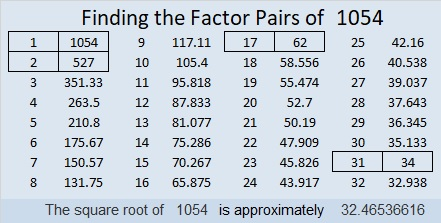1054 is the sum of six consecutive prime numbers:
163 + 167 + 173 + 179 + 181 + 191 = 1054

1054 is the hypotenuse of a Pythagorean triple:
496-930-1054 which is (8-15-17) times 62

1054 looks interesting when it is written in some other bases:
It’s 4A4 in BASE 15 (A is 10 base 10) because 4(15²) + 10(15) + 4(1) = 1054
1C1 in BASE 27 (C is 12 base 10) because 27² + 12(27) + 1 = 1054
VV in BASE 33 (V is 31 base 10) because 31(33) + 31(1) = 31(34) = 1054
V0 in BASE 34 because 31(34) = 1054

# What Kind of Shape is 976 in?

If you look at a list of Centered Triangular Numbers, 976 will be the 26th number on the list. 976 is the sum of the 24th, 25th, and 26th triangular numbers. That means
(24×25 + 25×26 + 26×27)/2 = 976

There’s a formula to compute centered triangular numbers, and this one is found by using
(3(25²) + 3(25) + 2)/2 = 976

As far as the formula is concerned, it is the 25th centered triangular number even though it is the 26th number on the list. I’m calling it the 26th centered triangular number because counting numbers on a list is easier than using a formula.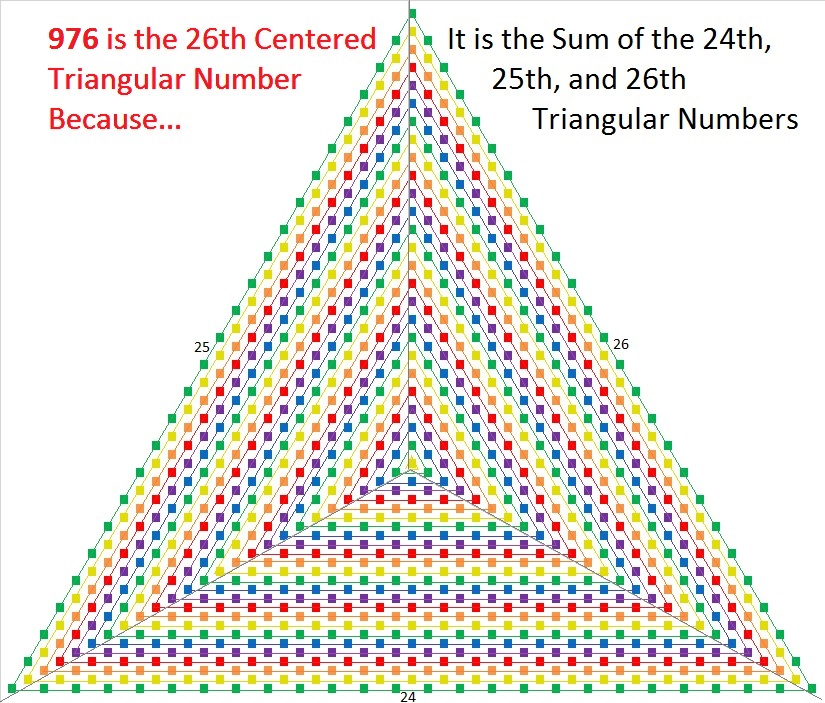976 is also the 16th decagonal number because 4(16²) – 3(16) = 976. I couldn’t resist illustrating that 10-sided figure.16 × 61 is a palindromic expression that happens to equal 976.

976 is also a palindrome when written in some other bases:
It’s 1100011 in BASE 3 because 3⁶ + 3⁵ + 3¹ + 3⁰ = 976,
808 in BASE 11, because 8(11²) + 0(11¹) + 8(11⁰) = 976
1E1 in BASE 25 (E is 14 base 10) because 1(25²) + 14(25¹) + 1(25⁰) = 976

24² + 20² = 976 That makes 976 the hypotenuse of a Pythagorean triple:
176-960-976 calculated from 24² – 20², 2(24)(20), 24² + 20²

• 976 is a composite number.
• Prime factorization: 976 = 2 × 2 × 2 × 2 × 61, which can be written 976 = 2⁴ × 61
• The exponents in the prime factorization are 4 and 1. Adding one to each and multiplying we get (4 + 1)(1 + 1) = 5 × 2 = 10. Therefore 976 has exactly 10 factors.
• Factors of 976: 1, 2, 4, 8, 16, 61, 122, 244, 488, 976
• Factor pairs: 976 = 1 × 976, 2 × 488, 4 × 244, 8 × 122, or 16 × 61
• Taking the factor pair with the largest square number factor, we get √976 = (√16)(√61) = 4√61 ≈ 31.240999# 901 and Level 4Print the puzzles or type the solution in this excel file: 10-factors-897-904

901 is the 25th centered triangular number because (23×24 + 24×25 + 25×26)/2 = 901. That is the same as saying that 901 is the sum of the 23rd, the 24th, and the 25th triangular numbers.

901 is the sum of two squares two different ways:

• 30² + 1² = 901
• 26² + 15² = 901

901 is the hypotenuse of FOUR Pythagorean triples:

• 60-899-901, calculated from 2(30)(1), 30² – 1², 30² + 1²
• 424-795-901, which is (8-15-17) times 53
• 451-780-901, calculated from 26² – 15², 2(26)(15), 26² + 15²
• 476-765-901, which is 17 times (28-45-53)

Two of those were primitives. That can only happen because ALL of 901’s prime factors are Pythagorean triple hypotenuses.

• 901 is a composite number.
• Prime factorization: 901 = 17 × 53
• The exponents in the prime factorization are 1 and 1. Adding one to each and multiplying we get (1 + 1)(1 + 1) = 2 × 2 = 4. Therefore 901 has exactly 4 factors.
• Factors of 901: 1, 17, 53, 901
• Factor pairs: 901 = 1 × 901 or 17 × 53
• 901 has no square factors that allow its square root to be simplified. √901 ≈ 30.016662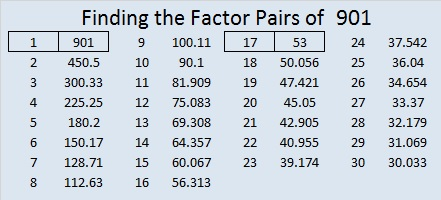# 694 and Level 6

• 694 is a composite number.
• Prime factorization: 694 = 2 x 347
• The exponents in the prime factorization are 1 and 1. Adding one to each and multiplying we get (1 + 1)(1 + 1) = 2 x 2 = 4. Therefore 694 has exactly 4 factors.
• Factors of 694: 1, 2, 347, 694
• Factor pairs: 694 = 1 x 694 or 2 x 347
• 694 has no square factors that allow its square root to be simplified. √694 ≈ 26.34388.Level 6 puzzles aren’t easy, but do give it a try! There are hints at the bottom of the page.Print the puzzles or type the solution on this excel file: 10 Factors 2015-11-23

———————————————————————————

Here are some ways that 694 is a special number:

694 is the 22nd centered triangular number because it is the sum of these consecutive triangular numbers 210, 231, 253.

Notice that 21(22)/2 is 231, the number in the middle of those consecutive triangular numbers. Also notice that 3(231) + 1 = 694.

694 BASE 10 becomes palindrome 1C1 in BASE 21 because C Base 21 is equivalent to 12 Base 10, and 1(21²) + 12(21) + 1(1) = 694.

———————————————————————————# 631 and Level 3

631 is a prime number that happens to also be the sum of the eleven prime numbers from 37 to 79.

Sometimes mathematicians talk about triangular numbers, centered triangular numbers, hexagonal numbers, and centered hexagonal numbers. Is there any relationship between those terms?

• The sum of any three consecutive triangular numbers is a centered triangular number.
• ALL hexagonal numbers are triangular numbers.
• Most centered hexagonal numbers are NOT centered triangular numbers.

In my last post, I pointed out that 630 is a triangular number and a hexagonal number.

Well, amazingly the next number, 631, is a centered triangular number AND a centered hexagonal number.

How often does one more than a triangular number equal a centered triangular number? Also how often does one more than a hexagonal number equal a centered hexagonal number?

Let’s compare a couple of lists of these types of numbers up to 631. Red means a number is in both lists. Blue means the number in the 1st list is one less than the number in the 2nd list.

• Triangular numbers (with hexagonal numbers in bold type):
• 1, 3, 6, 10, 15, 21, 28, 36, 45, 55, 66, 78, 91, 105, 120, 136, 153, 171, 190, 210, 231, 253, 276, 300, 325, 351, 378, 406, 435, 465, 496, 528, 561, 595, 630.
• Centered triangular numbers (with numbers that are also centered hexagonal numbers in bold type):
• 1, 4, 10, 19, 31, 46, 64, 85, 109, 136, 166, 199, 235, 274, 316, 361, 409, 460, 514, 571, 631.

1 is the only number in the lists that is a triangular, centered triangular, hexagonal, and centered hexagonal number all at the same time. (1 is also a square number, a pentagonal number, etc.)

(630, 631) is the first combination of numbers that has BOTH a triangular number immediately followed by a centered triangular number AND a hexagonal number immediately followed by a centered hexagonal number.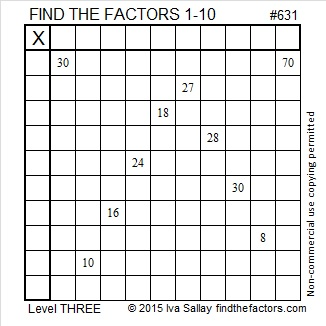Print the puzzles or type the solution on this excel file: 10 Factors 2015-09-28

—————————————————————————————————

• 631 is a prime number.
• Prime factorization: 631 is prime.
• The exponent of prime number 631 is 1. Adding 1 to that exponent we get (1 + 1) = 2. Therefore 631 has exactly 2 factors.
• Factors of 631: 1, 631
• Factor pairs: 631 = 1 x 631
• 631 has no square factors that allow its square root to be simplified. √631 ≈ 25.11971.How do we know that 631 is a prime number? If 631 were not a prime number, then it would be divisible by at least one prime number less than or equal to √631 ≈ 25.1. Since 631 cannot be divided evenly by 2, 3, 5, 7, 11, 13, 17, 19, or 23, we know that 631 is a prime number.

—————————————————————————————————

A Logical Approach to solve a FIND THE FACTORS puzzle: Find the column or row with two clues and find their common factor. (None of the factors are greater than 10.)  Write the corresponding factors in the factor column (1st column) and factor row (top row).  Because this is a level three puzzle, you have now written a factor at the top of the factor column. Continue to work from the top of the factor column to the bottom, finding factors and filling in the factor column and the factor row one cell at a time as you go.